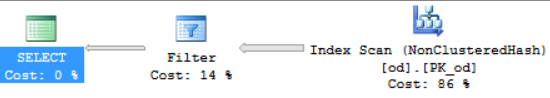In-Memory OLTP introduces a new type of index for memory-optimized tables in SQL Server 2014: the hash index. This new index type is optimized for index seek operations, i.e. finding the rows corresponding to a given set of equality predicates; for example, ‘o_id=8 AND od_id=4’. The underlying data structure of a hash index is a hash table, which is quite different from the B-tree data structure underlying the traditional (non)clustered indexes for disk-based tables.

In this post we will not describe the hash index structures in details; more information can be found in the Books Online topic on In-Memory OLTP. Instead, we focus on troubleshooting two common issues we have seen with customers using hash indexes. For each of the common issues we describe how to troubleshoot and how to work around the issue. Future blog posts will address further common performance problems.

# Hash index bucket count too low

Issue: If the bucket count is significantly lower (think 10X) than the number of unique index keys, there will be many buckets that have multiple index keys. This degrades performance of most DML operations, in particular point lookups, i.e. lookups of individual index keys.

Symptom: A performance degradation of queries that rely on lookups or inserts into the hash index. For example, SELECT queries and UPDATE/DELETE operations with equality predicates matching the index key columns in the WHERE clause.

How to troubleshoot: In some cases the problem is obvious from the index definition and the table data. For example, if the PRIMARY KEY has a HASH index with bucket_count 10,000, and the table has 1,000,000 rows, the bucket count is too low and will need to be changed.

In addition to inspecting table schema and data, you can use the DMV sys.dm_db_xtp_hash_index_stats. You can use the following query to obtain statistics concerning the buckets, and the row chains hanging off the buckets:

`SELECT hs.object_id, object_name(hs.object_id) AS 'object name', i.name as 'index name', hs.*FROM sys.dm_db_xtp_hash_index_stats AS hs JOIN sys.indexes AS i ON hs.object_id=i.object_id AND hs.index_id=i.index_id;`

A large average chain length indicates that many rows are hashed to the same bucket. If, in addition, the number of empty buckets is low or the average and maximum chain lengths are similar, it is likely that the total bucket count is too low. In this case, you need to increase the bucket_count. Typically, you would want the bucket_count to be between 1 and 2 times the number of unique index key values. Note that the bucket_count is automatically rounded up to the nearest power of 2.

# Search requires a subset of hash index key columns

Issue: Hash indexes require values for all index key columns in order to compute the hash value, and locate the corresponding rows in the hash table. Therefore, if a query includes equality predicates for only a subset of the index keys in the WHERE clause, SQL Server cannot use an index seek to locate the rows corresponding to the predicates in the WHERE clause.

In contrast, ordered indexes like the traditional disk-based (non)clustered indexes and the new memory-optimized nonclustered indexes (to be introduced in CTP2) support index seek on a subset of the index key columns, as long as they are the leading columns.

Symptom: This results in a performance degradation, as SQL Server will need to execute full table scans, rather than an index seek, which is typically a far cheaper operation.

How to troubleshoot: Besides the performance degradation, inspection of the query plans will also show a scan instead of an index seek. If the query is fairly simple, inspection of the query text and index definition will also show whether the search requires a subset of the index key columns.

Consider the following table and query:

``create table dbo.od ( o_id int not null, od_id int not null, p_id int not null,constraint PK_od primary key nonclustered hash (o_id,od_id) with (bucket_count=10000) ) with (memory_optimized=on)select p_idfrom dbo.odwhere o_id=1``

The table has a hash index on the two columns (o_id, od_id), while the query has an equality predicate on (o_id). As the query has equality predicates on only a subset of the index key columns, SQL Server cannot perform an index seek operation using PK_od; instead, SQL Server has to revert to a full index scan, as shown in the following query plan visualization:Workarounds: There are a number of possible workarounds. For example:

• Re-create the index as type ‘nonclustered’ [available in CTP2] instead of ‘nonclustered hash’. The memory-optimized nonclustered index is ordered, and thus SQL Server can perform an index seek on the leading index key columns. The resulting primary key definition for the example would be:
``constraint PK_od primary key nonclustered``
• Change the current index key to match the columns in the WHERE clause.
• Add a new hash index that matches with the columns in the WHERE clause of the query. In the example, the resulting table definition would look at follows:
``create table dbo.od ( o_id int not null, od_id int not null, p_id int not null,constraint PK_od primary key nonclustered hash (o_id,od_id) with (bucket_count=10000), index ix_o_id nonclustered hash (o_id) with (bucket_count=10000) ) with (memory_optimized=on)``

Note that memory-optimized hash indexes do not perform optimally if there are a lot of duplicate rows for a given index key value: in the example, if the number of unique values for the column o_id is much smaller than the number of rows in the table, it would not be optimal to add an index on (o_id); instead, changing the type of the index PK_od from hash to nonclustered would be the better solution.